Next: Conclusions Up: The ACTION Network Previous: Active Memory

## Attention

It was pointed out in Bapi et al.  that it is possible to use the ACTION network to achieve attentional control. That will be explored more fully here analytically. The experiment being analysed is that of Chelazzi et al.  in which a monkey is trained to make an eye movement to a target (such as a square) in an array of similar shapes, of which the target had been seen a brief period earlier, but then removed while the monkey holds to the fixation point until the appearance of the array. Neuronal responses varied; for a neuron whose receptive field contained the target, the response was one of increase on appearance of the array, until the eye movement occurred. For a neuron whose receptive field did not contain the target, but another shape in the array, the neuron's activity first grew, but then decreased, as if suppressed by the first neuron whose receptive field contained the target. The relevant neuronal activities can be modelled by an ACT network which, to simplify the analysis, will be taken as composed of only two neurons. Again, to simplify, the value of n will be taken as 1. Then the equations for the two sets of neurons in the ACT net will be:where the two sets of neurons are denoted by the unprimed and primed variables respectively, and w is the strength of the lateral inhibition between them (the matrix elements ofin equation (27)).

In order to analyse how this coupled system could explain the results of Chelazzi et al. , a simplified version will be taken in which the function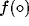is replaced by its linearised form, but the function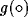is kept to be non-linear. After some manipulation, and use of the time shifting operator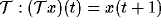and its inverse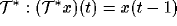, it is possible to obtain the coupled set of equations for the asymptotic behaviour of x and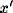as: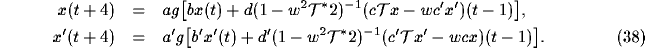To first order in the lateral interaction w between the two sets of unprimed and primed variables the equations (38) become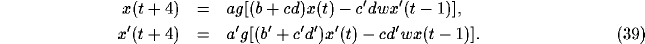By suitable change of the constants, these may be reduced to the pair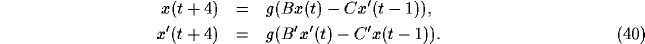The asymptotic values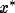,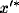of the activities x,therefore satisfy the equations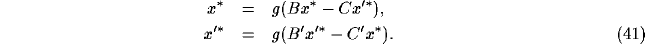It is possible to see that these have a solutionclose to 1 andclose to 0 if B>>C and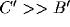. Since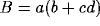,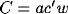,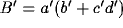and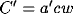, these inequalities are satisfied provided that, for example,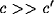. This corresponds to the learning of the connection from the cortex to the striatum for the undashed input, and will lead to the same qualitative behaviour in time for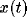and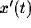as observed in the activities of the temporal lobe neurons of Chelazzi et al. . An important feature of the results of that reference is the form of the continued activity in the delay period of the relevant neurons in IT. If the activity is persistent then the parameters in (41) must allow for this; if not, then the activity will ultimately die away. Either case can be included in the above analysis, but nothing can be concluded until the data is clarified as to this point (for example, what length of delay can the subjects hold the memory for?).Next: Conclusions Up: The ACTION Network Previous: Active Memory